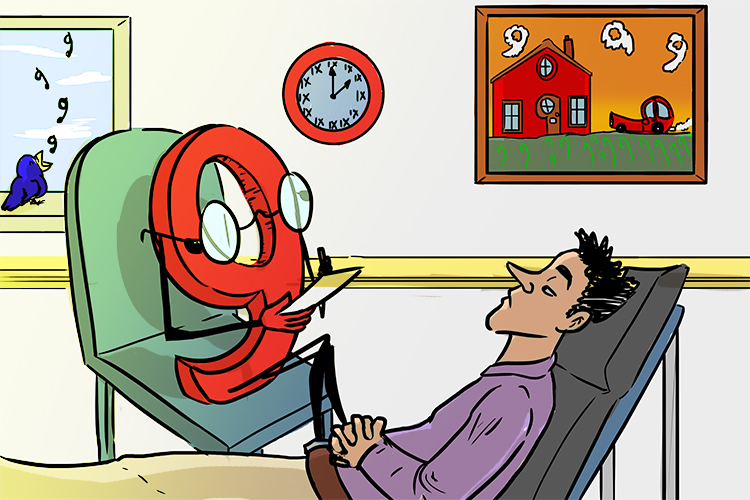# Decimal to fraction - Repeating

If you have a repeating decimal it is the division of any number by 9 that makes it repeat.

i.e.   1div9=1/9=0.111dot1

2div9=2/9=0.222dot2

3div9=3/9=0.333dot3I keep seeing nines everywhere doctor.

For repeating decimals think

 0 * 1 1 1 1 1 etc darr darr darr darr darr 1/9 1/90 1/900 1/9000 1/(90,000)

Or

 0 * 2 2 2 2 2 etc darr darr darr darr darr 2/9 2/90 2/900 2/9000 2/(90,000)

Or

 0 * 3 3 3 3 3 etc darr darr darr darr darr 3/9 3/90 3/900 3/9000 3/(90,000)

Find where it starts repeating and the number it repeats

Example 1

Convert 0.dot2 to a fraction

0.dot2=0.222222 recurring.

Remember:

 0 * 2 2 2 2 darr 2/9

Therefore

0.dot2=2/9

Now check on a calculator 2div9=0.22dot2

Example 2

Convert 0.0dot2 to a fraction

0.0dot2=0.0222222 recurring.

Remember:

 0 * 2 2 darr darr 2/9 2/90

Therefore

0.0dot2=2/90=1/45

Now check on a calculator 2div90=0.022dot2

Example 3

Convert 0.000222dot2 to a fraction

0.000dot2=0.000222222 recurring.

Remember:

 0 * 2 2 2 2 darr darr darr darr 2/9 2/90 2/900 2/(9,000)

Therefore

0.dot2=2/(9,000)=1/(4,500)

Now check on a calculator 2div9,000=0.00022dot2